Courses

# Test: Basic Concepts Of Differential And Integral Calculus- 1

## 40 Questions MCQ Test Business Mathematics and Logical Reasoning & Statistics | Test: Basic Concepts Of Differential And Integral Calculus- 1

Description
This mock test of Test: Basic Concepts Of Differential And Integral Calculus- 1 for CA Foundation helps you for every CA Foundation entrance exam. This contains 40 Multiple Choice Questions for CA Foundation Test: Basic Concepts Of Differential And Integral Calculus- 1 (mcq) to study with solutions a complete question bank. The solved questions answers in this Test: Basic Concepts Of Differential And Integral Calculus- 1 quiz give you a good mix of easy questions and tough questions. CA Foundation students definitely take this Test: Basic Concepts Of Differential And Integral Calculus- 1 exercise for a better result in the exam. You can find other Test: Basic Concepts Of Differential And Integral Calculus- 1 extra questions, long questions & short questions for CA Foundation on EduRev as well by searching above.
QUESTION: 1

### Choose the most appropriate option (a) (b) (c) or (d) The gradient of the curve y = 2x3 –3x2 – 12x +8 at x = 0 is

Solution:

Solution :- 2x^3 - 3x^2 -12x + 8 = 0

dy/dx = 6x^2 - 6x -12 = 0

(At x=0) = 6(0)^2 - 6(0) - 12

= -12

QUESTION: 2

Solution:
QUESTION: 3

### The derivative of y =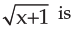Solution:
QUESTION: 4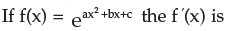Solution:
QUESTION: 5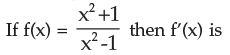Solution:
QUESTION: 6

If y = x (x –1) (x – 2) then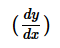is

Solution:
QUESTION: 7

The gradient of the curve y – xy + 2px + 3qy = 0 at the point (3, 2 ) is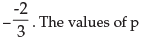and q are

Solution: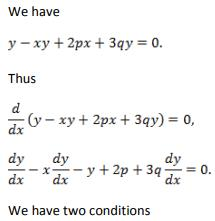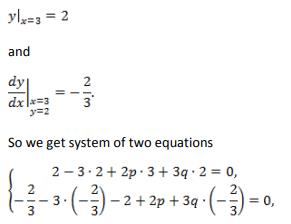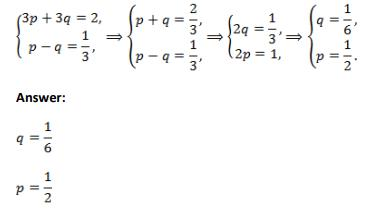QUESTION: 8

The curve y2 = ux3 + v passes through the point P(2, 3) and= 4 at P. The values of u and v are

Solution:
QUESTION: 9

The gradient of the curve y + px +qy = 0 at (1, 1) is 1/2. The values of p and q are

Solution:
QUESTION: 10

If xy = 1 then y2 + dy/dx is equal to

Solution:
QUESTION: 11

The derivative of the function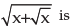Solution:
QUESTION: 12

Given e-xy –4xy = 0,can be proved to be

Solution:
QUESTION: 13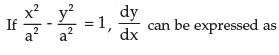Solution:
QUESTION: 14

If log (x / y) = x + y,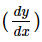may be found to be

Solution:
QUESTION: 15

If f(x, y) = x3 + y3 – 3axy = 0,can be found out as

Solution:
QUESTION: 16

Given x = at2, y = 2at;is calculated as

Solution:
QUESTION: 17

Given x = 2t + 5, y = t2 – 2;is calculated as

Solution:
QUESTION: 18

If y =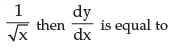Solution:
QUESTION: 19

If x = 3t2 –1, y = t3 –t, thenis equal to

Solution:
QUESTION: 20

The slope of the tangent to the curve y =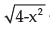at the point, where the ordinate and the abscissa are equal, is

Solution:
QUESTION: 21

The slope of the tangent to the curve y = x2 –x at the point, where the line y = 2 cuts the curve in the Ist quadrant, is

Solution:
QUESTION: 22

For the curve x2 + y2 + 2gx + 2hy = 0, the value ofat (0, 0) is

Solution:
QUESTION: 23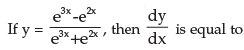Solution:
QUESTION: 24

If xy.yx = M, M is constant thenis equal to

Solution:
QUESTION: 25

Given x = t + t–1 and y = t – t–1 the value ofat t = 2 is

Solution:
QUESTION: 26

If x3 –2x2 y2 + 5x +y –5 =0 thenat x = 1, y = 1 is equal to

Solution:
QUESTION: 27

The derivative of x2 log x is

Solution:
QUESTION: 28

The derivative of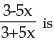Solution:
QUESTION: 29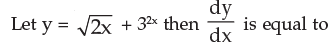Solution:
QUESTION: 30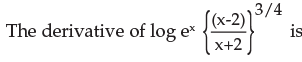Solution:
QUESTION: 31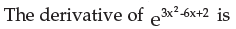Solution:
QUESTION: 32

If f(x) = 3x2, then F(x) =

Solution:
QUESTION: 33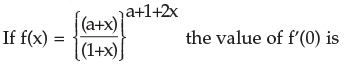Solution:
QUESTION: 34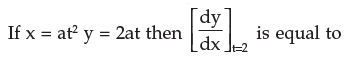Solution:
QUESTION: 35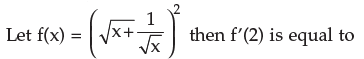Solution:
QUESTION: 36

If f(x) = x2 – 6x+8 then f ’(5) – f ’(8) is equal to

Solution:
QUESTION: 37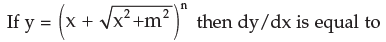Solution:
QUESTION: 38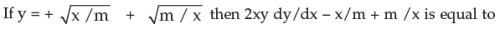Solution:
QUESTION: 39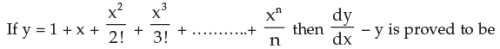Solution:
QUESTION: 40

If f(x) = xk and f’(1) = 10 the value of k is

Solution: